Practice the questions of McGraw Hill Math Grade 4 Answer Key PDF Chapter 10 Test to secure good marks & knowledge in the exams.

Place a check mark next to the best answer.

Question 1.
About how long is a paperclip?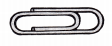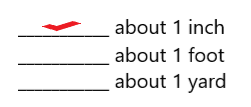Explanation:
The paperclip is about 1 inch long.

Question 2.
About how long is a horse?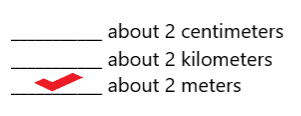Explanation:
The horse is about 2 meters long.

Multiply to find each missing number.

Question 3.
3 yd = ___________ ft
3 yd = 9 feet

Explanation:
1 yard = 3 feet
3 yards = 3 x 3 = 9 ft
So, 3 yd = 9 ft.

Question 4.
5 mi = ___________ yd
5 mi = 8800 yd

Explanation:
1 mile = 1760 yd
5 miles = 5 x 1760 = 8800 yd
So, 5 miles = 8800 yards.

Question 5.
4 yd = ___________ in
4 yd = 144 in

Explanation:
1 yard = 36 inches
4 yards = 4 x 36 = 144 in
So,4 yd = 144 in.

Question 6.
13 m = ___________ cm
13 m = 1300 cm

Explanation:
1 m = 100 cm
13 m = 13 x 100 cm
1300 cm
So, 13 cm = 1300 cm

Question 7.
48 km = ___________ m
48 km = 48000 m

Explanation:
1 km = 1000 m
48 km = 48 x 1000 m
= 48000 m
So, 48 km = 48000 m.

Question 8.
3 mi = ___________ ft
3 mi = 15,480 ft

Explanation:
1 mi = 5,280 ft
3 mi = 3 x 5280 ft
= 15,480 ft
So, 3 mi = 15,480 ft.

Question 9.
11 yd = ___________ in
11 yd = 396 in

Explanation:
1 yard = 36 inches
11 yards = 11 x 36 =  in
So,11 yd = 396 in.

Question 10.
17 m = ___________ cm
17m = 1700 cm

Explanation:
1 m = 100 cm
17 m = 17 x 100 cm
= 1700 cm
So, 17 m = 1700cm.

Question 11.
5 mi = ___________ ft
5 mi = 26,400 ft

Explanation:
1 mi = 5280 ft
5 mi = 5 x 5280 ft
26,400
So, 5 mi = 26,400 ft.

Question 12.
Draw a table to show the following data. Then convert each length to inches. Show both lengths in the table.
Maple Tree: 32 feet
Oak Tree: 75 feet
Birch Tree: 56 feet
Pine Tree: 95 feetExplanation:
I drew a table to show the given data
1 feet = 12 inches
So, i converted each length from feet to inches.

Question 13.
Draw a table to show the following data. Then convert each length to centimeters. Show both lengths in the table.
Height of Garden Shed: 3 meters
Height of Garage: 4 meters
Height of House: 22 meters
Height of Mailbox: 1 meter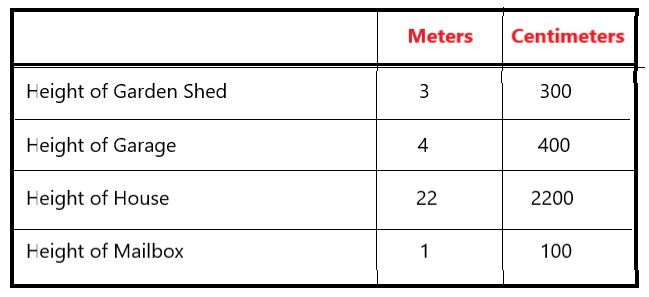Explanation:
I drew a table to show the given data
1 meter = 100 centimeters
So, i converted each length from meters to centimeters.

Question 14.
What is the area of the rectangle?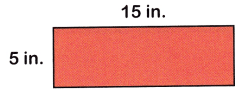75 square inches

Explanation:
Area of a rectangle = length x width
Length of the given figure = 15 in
Width of the given figure = 5 in
Area – l x w = 15 x 5 = 75
So, the area of the given figure is 75 square inches.

Question 15.
What is the area of the square?169 square meters

Explanation:
Area of a square = length x width
Length of the given figure = 13 m
Width of the given figure = 13 m
Area – l x w = 13 x 13 = 169
So, the area of the given figure is 169 square meters.

Question 16.
What is the perimeter of the rectangle?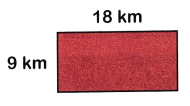54 kilometers

Explanation:
Perimeter of a rectangle = (2 x length) + (2 x width)
Length of the given figure = 18 km
Width of the given figure = 9 km
Perimeter = (2 x l) + (2 x w)
= (2 x 18) + (2 x 9)
= 36 + 18
= 54
So, perimeter of the given rectangle is 54 kilometers.

Solve.

Question 17.
Lauren and her brother went hiking. They hiked 44$$\frac{3}{8}$$ kilometers along the Atlanta Trail. They also hiked 36$$\frac{2}{8}$$ kilometers along the Hopper Trail. How many kilometers did they hike in all?
80$$\frac{5}{8}$$

Explanation:
Lauren and her brother went hiking
They hiked 44$$\frac{3}{8}$$ kilometers along the Atlanta Trail
They also hiked 36$$\frac{2}{8}$$ kilometers along the Hopper Trail
44$$\frac{3}{8}$$ + 36$$\frac{2}{8}$$ = 80$$\frac{5}{8}$$
They hike 80$$\frac{5}{8}$$ kilometers in all.

Question 18.
A fabric store has a sale. 22$$\frac{3}{5}$$ yards of black fabric are sold the first day and 19$$\frac{1}{5}$$ yards are sold the second day. 16$$\frac{1}{5}$$ yards of black fabric are leftover. How many yards of black fabric were in the store to begin with?
58 yards

Explanation:
A fabric store has a sale
22$$\frac{3}{5}$$ yards of black fabric are sold the first day
19$$\frac{1}{5}$$ yards are sold the second day
16$$\frac{1}{5}$$ yards of black fabric are leftover
22$$\frac{3}{5}$$ + 19$$\frac{1}{5}$$ + 16$$\frac{1}{5}$$ = 58 yards
So, 58 yards of black fabric were in the store to begin.

Question 19.
Mr. Harrison is an architect. He designs a rectangular living room that is 8 meters wide and 11 meters long. What is the area of the living room?
88 square meters

Explanation:
Mr. Harrison is an architect
He designs a rectangular living room that is 8 meters wide and 11 meters long
Area of a rectangle = length x width
Length of the living room = 11 meters
Width of the living room = 8 meters
Area – l x w = 11 x 8 = 88
So, the area of the living room is 8 square meters

Question 20.
What is the perimeter of the living room?
38 meters

Explanation:
Perimeter of a rectangle = (2 x length) + (2 x width)
Length of the living room = 11 meters
Width of the living room = 8 meters
Perimeter = (2 x l) + (2 x w)
= (2 x 11) + (2 x 8)
= 22 + 16
= 38
So, perimeter of the living room is 38 meters.

Question 21.
Karen has a new roll of tape. She uses 3$$\frac{1}{6}$$ feet to tape one package. She uses 5$$\frac{2}{6}$$ feet to tape another package. Then she uses 4$$\frac{1}{6}$$ feet to tape a third package. She has 12$$\frac{2}{6}$$ feet of tape left over. How many feet of tape did she begin with?
She uses 3$$\frac{1}{6}$$ feet to tape one package
She uses 5$$\frac{2}{6}$$ feet to tape another package
Then she uses 4$$\frac{1}{6}$$ feet to tape a third package
She has 12$$\frac{2}{6}$$ feet of tape left over
3$$\frac{1}{6}$$ + 5$$\frac{2}{6}$$ + 4$$\frac{1}{6}$$ + 12$$\frac{2}{6}$$ = 25 feet